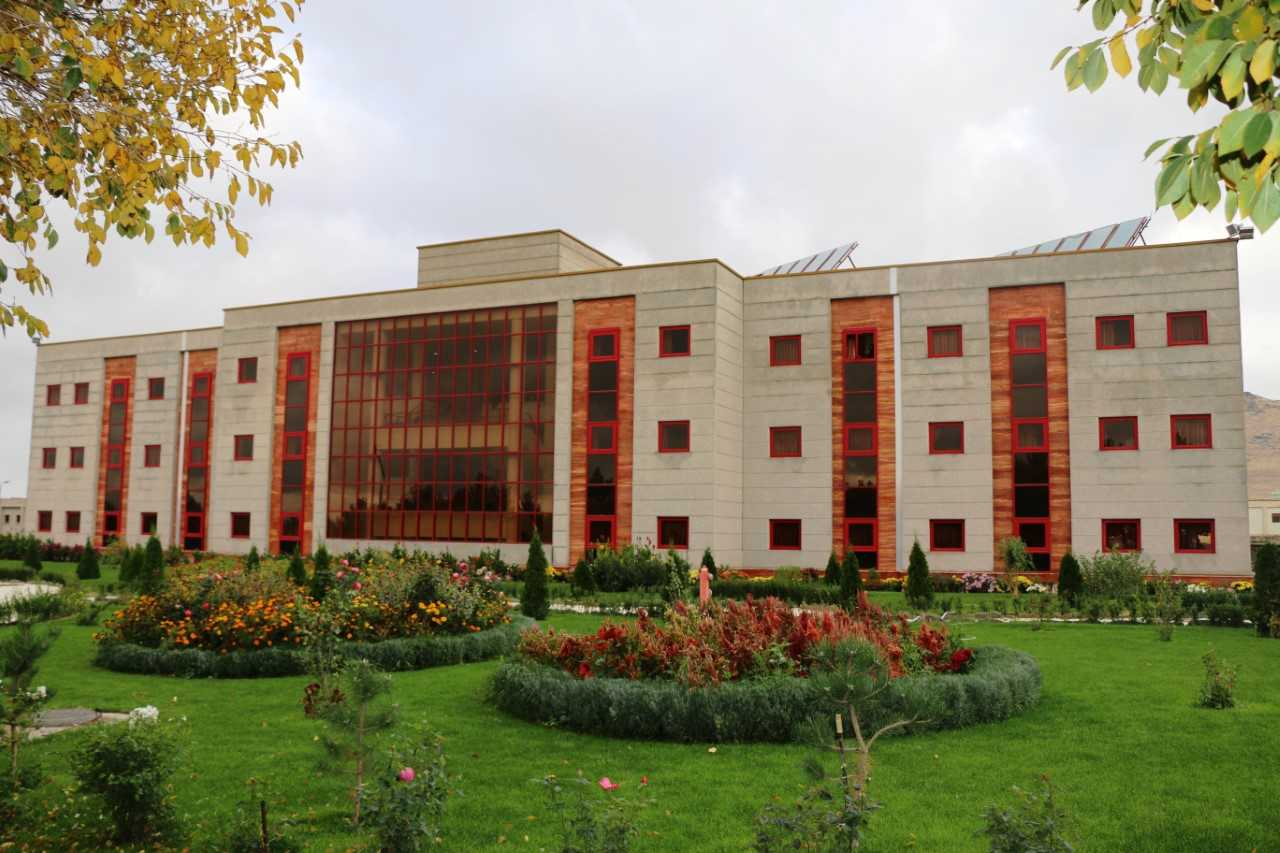CIMPA-IASBS Research School on Spectra in Riemannian and Symplectic Geometry
Zanjan, 4-15 July 2020One of the fascinating bridges between classical mechanics and quantum physics is expressed in the fact that the length spectrum and the Laplace spectrum of a closed Riemannian manifold determine each other (at least generically). Similarly, the set of lengths of the closed orbits of a billiard table and the Laplace spectrum of that table determine each other, at least for certain billiard tables. The main goal of this school is to introduce graduate students and young researchers to basic facts on these two spectra, and to the above correspondence. The length spectrum generalizes to the action spectrum of an autonomous Hamiltonian function. Floer homology filtered by the action spectrum can be used to construct so-called action selectors, that are a principal tool in modern symplectic geometry and dynamics, yielding simple proofs of Gromov's nonsqueezing theorem, the Weinstein conjecture in R^{2n}, the existence of closed orbits in a magnetic fields, etc. The second goal is to understand this relevance of the action spectrum in symplectic geometry. A third, more hypothetical, goal is to see which parts of the two sides can be related.

Prerequisites: We expect that students have had courses in real analysis of several variables, know what an ordinary differential equation is, and have followed a course on curves and surfaces. More knowledge is useful, but not necessary to follow the lectures.